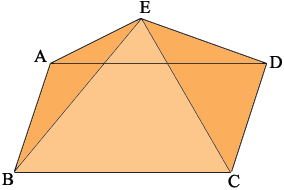SEARCH HOMEMath Central Quandaries & QueriesQuestion from Shivani, a student: What is the surface area of a rectangular pyramid with a length of 6 inches, a width of 7 inches and a SLANT height of 4 inches.Shivani,The base of the pyramid is a rectangle 6 inches by 7 inches so its area is 6 × 7 = 42 square inches. By the slant height do you mean |AE| = |BE| = |CE| = |DE|? If so then you know the length of the sides of each of the triangular faces. You can find the area of each of the triangles using Heron's Formula. The surface area is then the sum of the areas of the rectangular base and the four triangular sides.

PennyMath Central is supported by the University of Regina and The Pacific Institute for the Mathematical Sciences.